# Hydraulic Pumps Notes | Study Mechanical Engineering SSC JE (Technical) - Mechanical Engineering

## Mechanical Engineering: Hydraulic Pumps Notes | Study Mechanical Engineering SSC JE (Technical) - Mechanical Engineering

The document Hydraulic Pumps Notes | Study Mechanical Engineering SSC JE (Technical) - Mechanical Engineering is a part of the Mechanical Engineering Course Mechanical Engineering SSC JE (Technical).
All you need of Mechanical Engineering at this link: Mechanical Engineering

HYDRAULIC PUMPS

CENTRIFUGAL PUMP

• Centrifugal pump is reverse of inward flow reaction turbine. It works on princiepleof forced vortex motion. It has high discharging capacity and can be used for lifting highly viscous liquids e.g. sewage water, chemicals etc.
•  Priming is an operation in which liquid is completely filled in the chamber of pump so that air or gas or vapour from the portion of pump is driven out & no air pocket is left
• In volute pump cross sectional area results in developing a uniform velocity throughout the casing & free vortex is formed.
• Centrifugal pump has high output and high efficiency.
• Head Vs discharge and Power Vs discharge relationship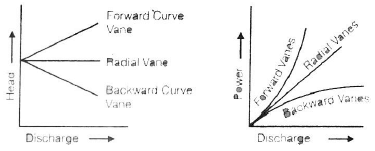•
•
 Pump Specific Speed Radial Flow 10 to 80 Mixed Flow 80 to 160 Axial Flow 160 to 450
•  work done persecond =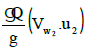(If discharge enters without whirl & shock, Vw1 = 0
• Static head (Hs) = hs + hd = static suction lift + static delivery lift
•  Manometric Head (Hm) it is the head against which pump has to do the work.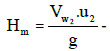Losses of head in the pump
(or)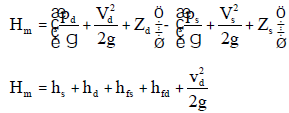where
pd & ps are pressures at delivery & suction points.
Vd & Vs are velocities at delivery & suction points.
Zd & Zs are position head of delivery & suction points.

• Efficiencies of the pump

(i) Manometric Efficiency (hman)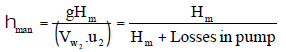(ii) Volumetric Efficiency (hvol)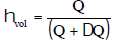(iii) Mechanical Efficiency (h mech)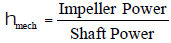(iv) Overall Efficiency (ho)
ho = h man × hvol × hmech

• The specific speed of a centrifugal pump may be defined as the speed in revolution per minute of a geometrically similar pump of such a size that under corresponding conditions it would deliver 1 litre of liquid per second against of a head of 1m.

For multi stage (Hm) =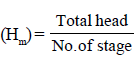Specific speed (Ns) =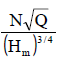• Pumps in Series : The pumps are connected in series in order to increase the head at constant discharge.

Q1 = Q2 = Q3 = ...
H = H1 + H+ H+ ...

Pumps in parallel :
The pumps are connected in parallel in order to increase the discharge at constant head.
Q = Q1 + Q2 + Q3 + ...
H = H1 = H2 = H3 = ...

•  Minimum speed needed to start the pumping :
If the pressure head raised is greater or equal to Hm, then pump will start pumping.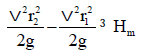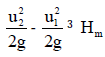• Speed ratio of pump ku =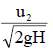= 0.95 to1.25
• Flow ratio of pump Y =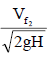= 0.1to 0.25
•  For optimum efficiency impeller should be designed such that whirl velocity at inlet is zero. It means discharge should enter in the pum radially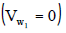• Model Laws in Pumps :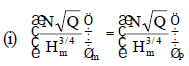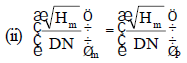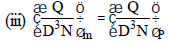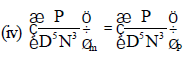D = Outer diameter of impeller
P = Shaft power
N = Impeller speed in rpm

Characterstic Curve of Pump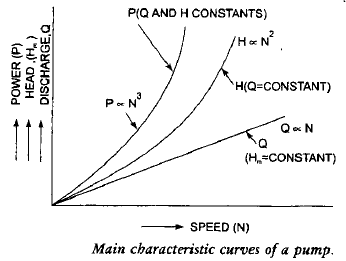Cavitation in pumps
The following factors contribute towards onset of cavitation.
(i) High runner speed
(ii) High temperature
(iii) Less available NPSH
For no cavitation, NPSH ³ scHs
c = Critical Thoma’s number  =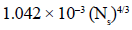where Ns is specific speed of pump.
NPSH = (Ha – hs) – Hv
where, Ha = atmospheric pressure head

RECIPROCATING PUMP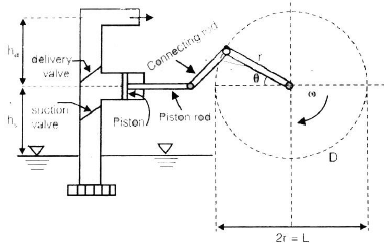Volume of water discharged per second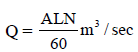A = Area of cylinder (in m2
L = Length of cylinder (in m)
N = Crank speed (in rpm)

•  If the head against which water is to be lifted is

Hs = (hs + hd)

• Work done per second =gQ(hs + hd), where g = dg
• Reciprocating pumps are used to lift water against high head at low discharge.
•  To increase discharge and to maintain it more uniform, double acting reciprocating pumps are used.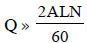thus power also gets doubled.

•  Slip in Percentage is given by

%slip =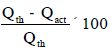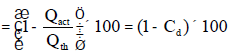where Cd = coefficient of discharge
Slip is negative when (i) delivery pipe is small and suction pipe is long (ii) Pump is running at very high speed.

• Indicator diagram is a graph between the pressure head in the cylinder and the distance travelled by the piston flow inner dead centre for one complete revolution of the crank, work done by pump is proportional to area of indicator diagram.
• Air Vessel is used to obtain continuous supply of water at uniform rate, to save a considerable amount of work and to run the pump at a high speed without separation.
•  Percentage of work saved is 84.8% when single acting pump with air vessel is used while this saving is only 39.2% when air vessel is used in double acting pump.
• Advantage of multicylinder pumps are that the pump even without air vessels deliver liquid more uniformly as compared to single cylinder pump.
• Operating Characteristic Curve of Reciprocating Pump is given below :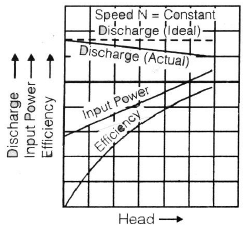The document Hydraulic Pumps Notes | Study Mechanical Engineering SSC JE (Technical) - Mechanical Engineering is a part of the Mechanical Engineering Course Mechanical Engineering SSC JE (Technical).
All you need of Mechanical Engineering at this link: Mechanical EngineeringUse Code STAYHOME200 and get INR 200 additional OFF

## Mechanical Engineering SSC JE (Technical)

6 videos|89 docs|55 tests

Track your progress, build streaks, highlight & save important lessons and more!

,

,

,

,

,

,

,

,

,

,

,

,

,

,

,

,

,

,

,

,

,

;